## Example Questions

2 Next →

### Example Question #21 : Exponents

Simplify: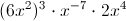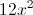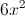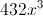Explanation:

Remember, we add exponents when their bases are multiplied, and multiply exponents when one is raised to the power of another. Negative exponents flip to the denominator (presuming they originally appear in the numerator).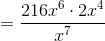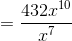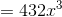### Example Question #471 : Algebrais a real number such that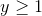.

Quantity A: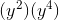Quantity B: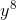The two quantities are equal.

Quantity A is greater.

Quantity B is greater.

The relationship cannot be determined from the information given.

The relationship cannot be determined from the information given.

Explanation:

(y2)(y4) = y2+4 = y6

Plug in two different values for y.

Plug in y = 1: y8 = y

Plug in y = 2: y8 > y

Since the results differ, the relationship cannot be determined from the information given.

### Example Question #13 : How To Multiply Exponents

Evaluate: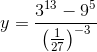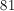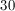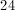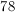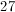Explanation:

Can be simplified to: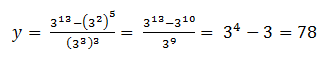### Example Question #14 : How To Multiply Exponents

Simplify the following expression.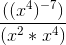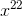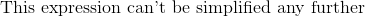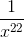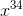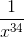Explanation:

To solve this problem, we must first understand some of the basic concepts of exponents. When multiplying exponents with the same base, one would simply add the powers together with the same base to obtain the result. For example,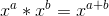.

When raising exponents to a certain power, one would simply multiply the power the exponent is being raised to with the exponent itself to obtain the new exponent. The base also gets raised to the same power as it normally would and the new exponent gets put on afterward. For example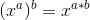.

Now that we have covered the basic concepts of exponent manipulation, we can now solve the problem. The top part of the expression give to us is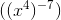. As we have stated before, when an exponent is raised to a certain power, you simply multiply the power and the exponent together. This results in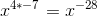.

The new expression becomes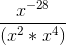.

Solving for the denominator of the equation, we previously stated that when multiplying exponents together with the same base, we simply add the exponents together and keep the same base. Therefore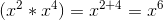.

The new expression becomes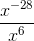, however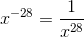, therefore the expression becomes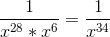.

2 Next →

Tired of practice problems?

Try live online GRE prep today.